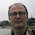## Pages

### HackerRank DFS: Connected Cell in a Grid

In this HackerRack problem, we are given in input an n x m matrix containing as elements just 0s and 1s. We should give as output the size of the largest available region.

As suggested in the name of the problem, we should think of a solution that refers to graphs, and more specifically on the Depth First Search algorithm on them.

To better understand what are we required to get, let's have a look at the provided example:
```[1, 1, 0, 0]
[0, 1, 1, 0]
[0, 0, 1, 0]
[1, 0, 0, 0]
```
This matrix has two so called "regions", one takes just the 1 in the bottom left corner, the other all the remaining ones. Obviously, the largest one is the latter, so we are expected to return 5.

Notice that two 'alive' cells are considered connected if they have distance one, horizontally, vertically, or diagonally.

Following the hint, I implemented my python solution applying the DFS on each cell in the matrix.
```def solution(matrix):
result = 0
for i in range(len(matrix)):
for j in range(len(matrix)):
result = max(result, connected(matrix, i, j))  # 1
return result
```
1. The result is initialized to zero, when connected() return a value higher than the current result, it takes the place of the current better solution found.

Now I just have to write the connected() function. Based on DFS, it would recursively call itself looking for all the cell connected to the current one. Slight variation, it would return the size of the group. For efficiency reasons, I decided to implement it in a disruptive way. The matrix would be modified to keep track of the visited cells. This is usually not a good idea, but in a problem like this, we can live with it.
```def connected(matrix, i, j):
if not (0 <= i < len(matrix)) or not (0 <= j < len(matrix)):
return 0  # 1

if matrix[i][j] != 1:
return 0  # 2

result = 1  # 3
matrix[i][j] = 0  # 4

for ii in range(i-1, i+2):
for jj in range(j-1, j+2):
if i != ii or j != jj:
result += connected(matrix, ii, jj)  # 5

return result
```
1. If we are stepping out the matrix borders, there's nothing to do. Just return zero.
2. If the current cell is not "alive", it is not part of any group, return zero.
3. Otherwise, count it for the current group.
4. This is the soft spot I talked about above. Instead of keeping track of the visited node, I simply set it to "dead". It won't harm to the algorithm, since we would count each node just once, still it could be surprising for the caller seeing its matrix changed.
5. Go and visit all the nodes connected to the current one. The two for-loops select the indices in the current cell adjacency, the "if" excludes the current one. We will check if the selected position refers to an actual connected node in (1) and (2) and then apply the recursion.

And that's it. Full python code and test case I used to help me getting the solution is on GitHub.

1.1.2.3.